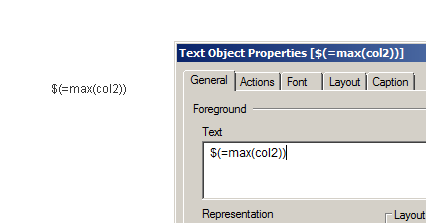# New to QlikView

Discussion board where members can get started with QlikView.

Contributor III

## What does this \$ mean?

Hi,

Looking at the below SUM statement,

sum(

{

<

col1={'1'},

col2 = {'\$(=max(col2))'},

col3 = {'YTD'}>

}

amount

)

when it's evaluated, looks like the end result of col2 may look something like col2 = '123'.  My question is about the \$ (dollar) sign

there.  Can someone explain to me what the \$ sign mean when it's used like this?

10 RepliesEmployee

## Re: What does this \$ mean?

Its an indication to the qlik engine resolve the expression between the \$() , replace with the resulting values, before evaluating the expression.

The Magic of Dollar ExpansionsMVP

## Re: What does this \$ mean?

dollar sign expansion

here

The Magic of Dollar Expansions

and in the Qlik help

## Dollar-Sign Expansion with an Expression

Expressions can be used in dollar-sign expansions. The content between the brackets must then start with an equal sign:

\$( =expression )

The expression will be evaluated and the value will be used in the expansion.

Example:

\$(=Year(Today())); // returns e.g. '2008'

\$(=Only(Year)-1); // returns the year before the selected one

Contributor III

## Re: What does this \$ mean?

Jonathan,

So is that the same thing as \$(vMyDirectory)?  Sounds like it. I was just confused when \$ is used inside

a pair of single tick marks.  So if I simply want a string of text to look exactly like \$(=max(col2)), what do I need to do?

Just out of curiosity.

maxgro.

What do you by "returns the year before the selected one"..  I am not following this definition.

ThanksEmployee

## Re: What does this \$ mean?

Well, dollar sign expansion is frequently used with variables but not always (as per your example).  vMyDirectory is most likely a variable and you can swap in a reusable expression to be evaluated WITHIN the context of the chart you are invoking the variable with this technique.  If the variable is just text like this with no preceding equals:   Max(col2)  then you will probably need the dollar sign expansion to have the text from the variable evaluated as an expression.

But if the text  has a preding equals sign:   =Max(col2)    ...then qlik will likely pre-evaluate it as an expression, calculated the value OUTSIDE the context of where you are using and just insert the value. In that case, you may not  need dollar sign expansion.

This can help with the subtleties

The Little Equals Sign

Contributor III

## Re: What does this \$ mean?

OK, this is clearer now.  So If I want my text to appear on the screen to look exactly like this: \$(=max(col2)), how do you do it?Employee

## Re: What does this \$ mean?

You mean like this ?Partner

## Re: What does this \$ mean?

Hi,

Just have the '\$(=max(col2))' in text box

Valued Contributor III

## Re: What does this \$ mean?

Dear Sidneylu,

"\$" it's called Dollar-Sign Expansion if you are using in Set Analysis Modifier section,

Example:

=Sum({<Year = {\$(=Max(Year)}>})

"\$" it's called Dollar-Sign Expansion if you are using with Variable,

Example:

\$(vPreviousMonth)

"\$" it's called Current Selection if you are using in Set Analysis Identifier section.

Example:

=Sum({\$<Year = {2015}>})

Kind regards,

Ishfaque Ahmed

Contributor III

## Re: What does this \$ mean?

Just a quick note to thank everyone who responded.

This is much clearer to me now.